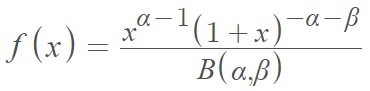# Beta Prime Distribution

Share on

Probability distributions > Beta prime distribution

The Beta Prime Distribution is a continuous probability distribution defined on the interval [0, ∞). The distribution has fat tails which decrease polynomially. In Bayesian statistics, the distribution is a conjugate family of prior distributions on the odds parameter of the binomial distribution.

The distribution has many other names, including: beta type II, compound gamma, gamma ratio, inverse (or inverted) beta, Pearson type VI, and variance ratio. The relationship between the beta distribution and beta prime is as follows: If a random variable Z is from a beta distribution, the X = Z-1 – 1 is from the beta prime distribution .

The standardized probability density function for the beta prime distribution isshape parameters and Β(α, β) is the beta function. Some definitions do include a scale parameter, λ, but this is not common; Most definitions are defined for λ = 1 .

The cumulative distribution function is: F(x) = Ix/(1+x)(α, β).

The mean is α(β – 1) for β > 1.
The mode is (α – 1)/ (α + β – 2) for α > 1, β > 1.
The median cannot be expressed in a simple closed form expression.

## Special Cases of the Generalized Beta Prime Distribution

The beta prime distribution has many special cases, overlapping with some families of probability distributions. For example, the power function distribution is a special case of the beta prime distribution when Β is negative. Other notable special cases include :

## References

 Fishman, G. (2001). Discrete-Event Simulation: Modeling, Programming, and Analysis. Springer.
 Laurent, S. (2019). R-Bloggers–The Beta Distribution of the Third Kind. Retrieved December 31, 2021 from: https://www.r-bloggers.com/2019/07/the-beta-distribution-of-the-third-kind-or-generalised-beta-prime/
 Crooks, G. Survey of Simple, Continuous, Univariate Probability Distributions. Retrieved December 31, 2021 from: https://citeseerx.ist.psu.edu/viewdoc/download?doi=10.1.1.372.3694&rep=rep1&type=pdf

CITE THIS AS:
Stephanie Glen. "Beta Prime Distribution" From StatisticsHowTo.com: Elementary Statistics for the rest of us! https://www.statisticshowto.com/beta-prime-distribution-2/
---------------------------------------------------------------------------Need help with a homework or test question? With Chegg Study, you can get step-by-step solutions to your questions from an expert in the field. Your first 30 minutes with a Chegg tutor is free!